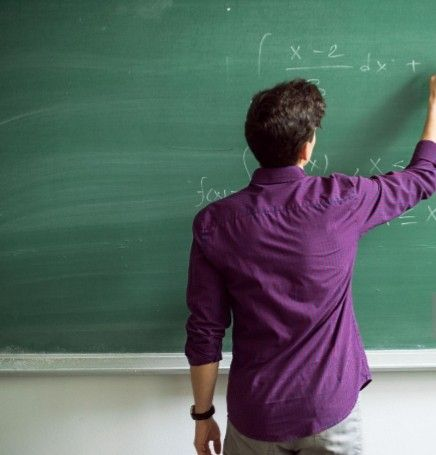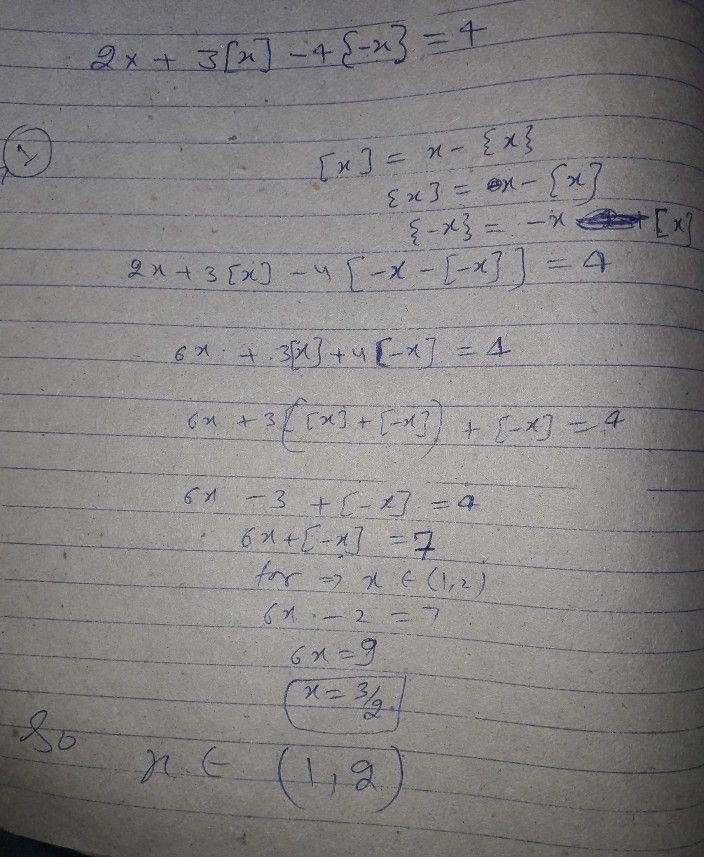Symbol
Problem$18.$ The solution of the equation $2x+3$ $\left(x\right)-4$ $\left(-x\right)$ $=4\left(whe$ (where [x] and $\left(\times 3$ denote integral and fractional part of $X\right)$ lies in the $inteWa1:$ $\left(A\right)$ $\left(0,1\right)$ (B) $\right)$ $\left(1,2\right)$ $\left(C\right)\left(2,3\right)$ (D) None of these
Other
SolutionQanda teacher - R.K SIRStudent
sir i can't understand
pls elaborateQanda teacher - R.K SIR
please tell me where you have problemStudent
where u take x belongs to 1,2Qanda teacher - R.K SIR
i will tell you in morningStudent
ok sir